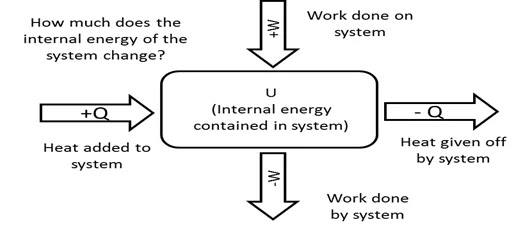20% Off Holiday Savings Sale Ends Today!* Use Promo Code: DECEMBER20 *Restrictions apply.

# Principles of Thermodynamics for Engineering Applications

• 27 February, 2017

Introduction

Thermodynamics is a branch of science that explains energy and its transformation based on the physical state of the matter. The analysis of thermal activities is derived by means of energy conservation equations, which are based on the conservation of mass or the conservation of energy. Thermodynamic principles mainly depend either on the law of conservation of energy or the law of conservation of mass. Law of conservation of mass and energy equations and calculations are thoroughly reviewed in our FE exam review courses.Thermodynamics Principles or Laws

Thermodynamics First Principle: The most important law of physics is the conservation of energy. The first law of thermodynamics states that energy can move from one physical state to another during molecular interaction, but the total energy remains the same and cannot be destroyed.

Thermodynamics Second Principle: The second law of thermodynamics states that the entropy of any independent or isolated system always increases, and the actual process of work moves in the direction of low energy quality.

Thermodynamics Third Principle: The third law of thermodynamics states that as the temperature reaches absolute zero, the entropy of a system tends to reach constant value. Therefore, the entropy of a system is zero at absolute zero temperature.

Thermodynamics Fourth Principle: The fourth law of thermodynamics states that if any two objects are in thermal equilibrium with the third object at the given time, then the heat transfer among the objects is "zero." This principle is often referred to as the "zeroth principle."

Working Principles of Thermodynamics

System: a predefined medium to analyze thermodynamic activities; the complexity depends on the nature of the industry. The work is based on the quantity of matter and shape of the system. The boundary surface of the system is the layer between the system and its surroundings.

Systems are classified as the following:

1)Open System: If material or matter can navigate through the boundary surface to its surroundings, then it is considered an open system.

2)Closed System: If material or matter cannot pass through the boundary surface to its surroundings, then it is said to be a closed system. Isolated systems are considered a type of closed system where there is no interaction with a material's surroundings.

The physical state of a system is defined by its properties and its values. The properties of a system change due to the working process of energy inputs and outputs during the period of transformation energy. Thermodynamic cycles have a series of processes that start and stop at the fixed state. It is necessary to understand the above-mentioned systems and laws for the Fundamentals of Engineering exam.

Energy Inputs and Outputs##### Blogs you might also like

Copied to clipboard